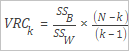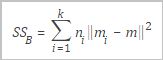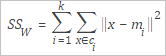The Clustering Model Evaluation component is used to evaluate clustering models and generate evaluation metrics based on raw data and clustering results.

## Background information

The Calinski-Harabasz index is also known as the variance ratio criterion (VRC). The following figure shows the formula used to calculate the VRC.Parameter Description
SSB The variance between clusters. The following figure shows the formula used to calculate the variance between clusters.Formula description:
• k: indicates the number of cluster center points.
• mi: indicates the center point of the cluster.
• m: indicates the mean value of the input data.
SSW The variance within a cluster. The following figure shows the formula used to calculate the variance within a cluster.Formula description:
• k: indicates the number of cluster center points.
• x: indicates data points.
• ci: indicates the ith cluster.
• mi: indicates the center point of the cluster.
N The total number of records.
k The number of cluster center points.

## Configure the component

You can configure the component by using one of the following methods:
• Use the Machine Learning Platform for AI console
Tab Parameter Description
Fields Setting Columns Included for Evaluation The columns that are selected from the input table for evaluation. The value of this parameter must be consistent with the feature columns in the model.
Input in Sparse Format Specifies whether the input data is in the sparse format. Data in the sparse format is presented by using key-value pairs.
KV Delimiter The delimiter that is used to separate key-value pairs. Commas (,) are used by default.
KV Delimiter The delimiter that is used to separate keys and values. Colons (:) are used by default.
Tuning Number of Cores The number of cores. This parameter must be used with the Memory Size per Core parameter. The value of this parameter must be a positive integer.
Memory Size per Core The memory size of each core. This parameter must be used with the Number of Cores. Unit: MB.
• Use commands
``````PAI -name cluster_evaluation
-project algo_public
-DinputTableName=pai_cluster_evaluation_test_input
-DselectedColNames=f0,f3
-DmodelName=pai_kmeans_test_model
-DoutputTableName=pai_ft_cluster_evaluation_out;``````
Parameter Required Description Default value
inputTableName Yes The name of the input table. N/A
selectedColNames No The names of the columns that are selected from the input table for evaluation. Separate multiple columns with commas (,). The value of this parameter must be consistent with the feature columns in the model. All columns
inputTablePartitions No The partitions that are selected from the input table for training. Specify this parameter in one of the following formats:
• Partition_name=value
• name1=value1/name2=value2: multi-level partitions
Note If you specify multiple partitions, separate these partitions with commas (,).
Full table
enableSparse No Specifies whether data in the input table is in the sparse format. Valid values: true and false. false
itemDelimiter No The delimiter that is used to separate key-value pairs in the sparse format. ,
kvDelimiter No The delimiter that is used to separate keys and values. :
modelName Yes The name of the input clustering model. N/A
outputTableName Yes The name of the output table. N/A
lifecycle No The lifecycle of the output table. N/A

## Example

1. Execute the following SQL statements to generate test data:
``````create table if not exists pai_cluster_evaluation_test_input as
select * from
(
select 1 as id, 1 as f0,2 as f3 from dual
union all
select 2 as id, 1 as f0,3 as f3 from dual
union all
select 3 as id, 1 as f0,4 as f3 from dual
union all
select 4 as id, 0 as f0,3 as f3 from dual
union all
select 5 as id, 0 as f0,4 as f3 from dual
)tmp;``````
2. Run the following PAI command to build a clustering model. A k-means clustering model is built in this example.
``````PAI -name kmeans
-project algo_public
-DinputTableName=pai_cluster_evaluation_test_input
-DselectedColNames=f0,f3
-DcenterCount=3
-Dloop=10
-Daccuracy=0.00001
-DdistanceType=euclidean
-DinitCenterMethod=random
-Dseed=1
-DmodelName=pai_kmeans_test_model
-DidxTableName=pai_kmeans_test_idx``````
3. Run the following PAI command to submit the parameters configured for the Clustering Model Evaluation component:
``````PAI -name cluster_evaluation
-project algo_public
-DinputTableName=pai_cluster_evaluation_test_input
-DselectedColNames=f0,f3
-DmodelName=pai_kmeans_test_model
-DoutputTableName=pai_ft_cluster_evaluation_out;``````
4. View the output evaluation table pai_ft_cluster_evaluation_out and the following visualized graph. The following table lists the mappings between the fields in the graph and those in the pai_ft_cluster_evaluation_out table.
Table Graph
count The total number of records.
centerCount The number of cluster centers.
calinhara The VRC metric.
clusterCounts The number of points included in each cluster.